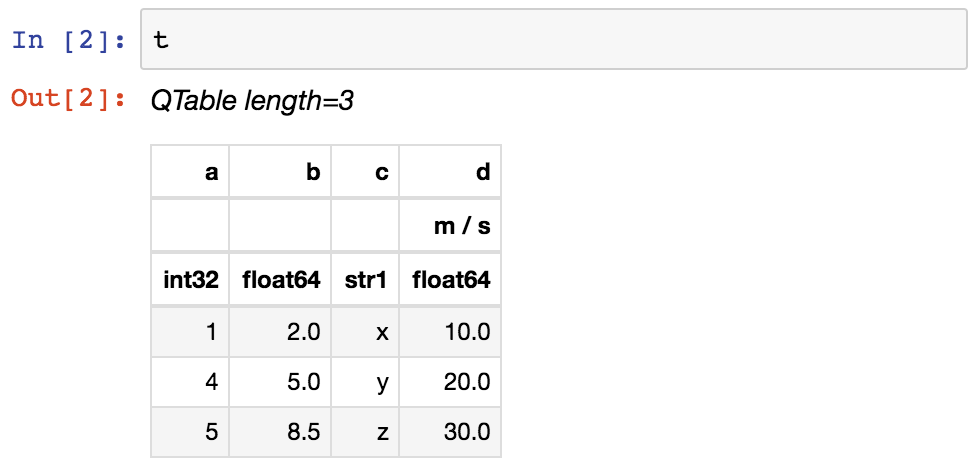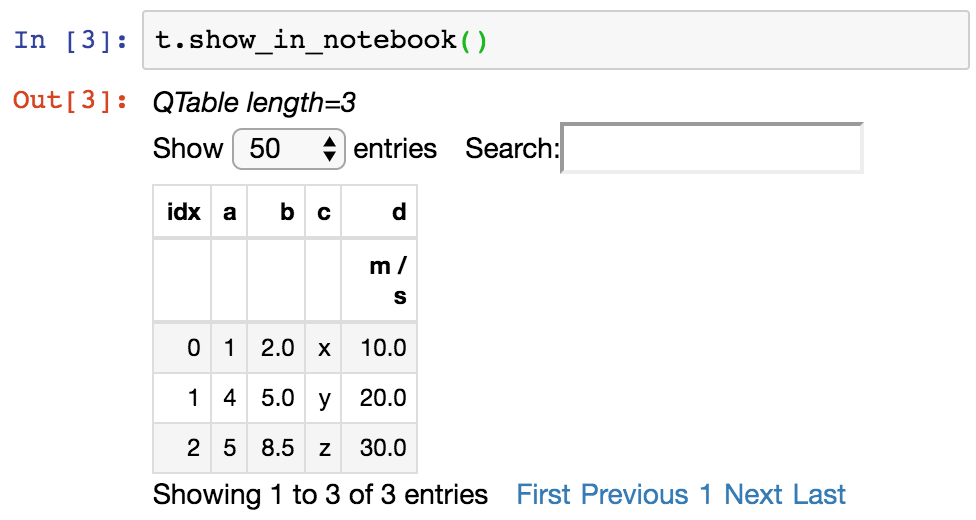# 数据表 (astropy.table ）¶

## 介绍¶

astropy.table 提供以熟悉的方式存储和操作异构数据表的功能 numpy 用户。该软件包的几个显著功能是：

• 从各种输入数据结构和类型初始化表。

• 通过添加或删除列、更改列名或添加新的数据行来修改表。

• 处理包含缺失值的表。

• 包括表和列元数据作为灵活的数据结构。

• 指定列的说明、单位和输出格式。

• 以交互方式滚动长表，类似于使用 more .

• 通过从表中选择行或列来创建新表。

• 表演 表操作 比如数据库连接、连接和binning。

• 维护表索引以快速检索表项或范围。

• 操纵多维列。

• 处理表中的非本机（mixin）列类型。

• 方法 读写表对象 到文件。

• 钩子 子类化表 及其组件类。

## 入门¶

>>> from astropy.table import QTable
>>> import astropy.units as u
>>> import numpy as np

>>> a = np.array([1, 4, 5], dtype=np.int32)
>>> b = [2.0, 5.0, 8.5]
>>> c = ['x', 'y', 'z']
>>> d = [10, 20, 30] * u.m / u.s

>>> t = QTable([a, b, c, d],
...            names=('a', 'b', 'c', 'd'),
...            meta={'name': 'first table'})


• a 是一个 numpy 使用指定的数组 dtype 属于 int32 . 如果未提供数据类型，则整数的默认类型为 int64 在Mac和Linux上 int32 在Windows上。

• b 是一个列表 float 值，表示为 float64 .

• c 是一个列表 str 值，以unicode表示。看到了吗 Bytestring列 更多信息。

• d 是一个 Quantity 数组。自从我们用过 QTable ，这将存储一个本机 Quantity 在桌子里，带来了 单位和数量 (astropy.units ） 到表中的此列。

>>> t
<QTable length=3>
a      b     c      d
m / s
int32 float64 str1 float64
----- ------- ---- -------
1     2.0    x    10.0
4     5.0    y    20.0
5     8.5    z    30.0


>>> t.info
<QTable length=3>
name  dtype   unit  class
---- ------- ----- --------
a   int32         Column
b float64         Column
c    str1         Column
d float64 m / s Quantity>>> print(t)
a   b   c    d
m / s
--- --- --- -----
1 2.0   x  10.0
4 5.0   y  20.0
5 8.5   z  30.0


>>> t['b'].info.format = '7.3f'
>>> print(t)
a     b     c    d
m / s
--- ------- --- -----
1   2.000   x  10.0
4   5.000   y  20.0
5   8.500   z  30.0


>>> t.more()


>>> t.show_in_browser()


>>> t.show_in_browser(jsviewer=True)


>>> t.colnames
['a', 'b', 'c', 'd']
>>> len(t)
3
>>> t.meta
{'name': 'first table'}


>>> t['a']       # Column 'a'
<Column name='a' dtype='int32' length=3>
1
4
5

>>> t['a']    # Row 1 of column 'a'
4

>>> t         # Row object for table row index=1
<Row index=1>
a      b     c      d
m / s
int32 float64 str1 float64
----- ------- ---- -------
4   5.000    y    20.0

>>> t['a']    # Column 'a' of row 1
4


>>> print(t[0:2])      # Table object with rows 0 and 1
a     b     c    d
m / s
--- ------- --- -----
1   2.000   x  10.0
4   5.000   y  20.0

>>> print(t['a', 'c'])  # Table with cols 'a', 'c'
a   c
--- ---
1   x
4   y
5   z


>>> t['a'][:] = [-1, -2, -3]    # Set all column values in place
>>> t['a'] = 30              # Set row 2 of column 'a'
>>> t = (8, 9.0, "W", 4 * u.m / u.s) # Set all row values
>>> t['b'] = -9              # Set column 'b' of row 1
>>> t[0:2]['b'] = 100.0         # Set column 'b' of rows 0 and 1
>>> print(t)
a     b     c    d
m / s
--- ------- --- -----
-1 100.000   x  10.0
8 100.000   W   4.0
30   8.500   z  30.0


>>> t['b'] = ['a', 'new', 'dtype']   # Replace column b (different from in-place)
>>> t['e'] = [1, 2, 3]               # Add column d
>>> del t['c']                       # Delete column c
>>> t.rename_column('a', 'A')        # Rename column a to A
>>> t.colnames
['A', 'b', 'd', 'e']


>>> t.add_row([-8, 'string', 10 * u.cm / u.s, 10])
>>> len(t)
4


>>> t = QTable([a, b, c], names=('a', 'b', 'c'), masked=True, dtype=('i4', 'f8', 'U1'))
>>> t['a'].mask = [True, True, False]
>>> t
<QTable masked=True length=3>
a      b     c
int32 float64 str1
----- ------- ----
--     2.0    x
--     5.0    y
5     8.5    z


>>> from astropy.time import Time
>>> from astropy.coordinates import SkyCoord
>>> tm = Time(['2000:002', '2002:345'])
>>> sc = SkyCoord([10, 20], [-45, +40], unit='deg')
>>> t = QTable([tm, sc], names=['time', 'skycoord'])
>>> t
<QTable length=2>
time          skycoord
deg,deg
object          object
--------------------- ----------
2000:002:00:00:00.000 10.0,-45.0
2002:345:00:00:00.000  20.0,40.0


>>> dt = t['time'] - Time('1999-07-23 04:30:59.984')
>>> t['dt_cxo'] = dt.to(u.d)
>>> t['dt_cxo'].info.format = '.3f'
>>> print(t)
time          skycoord   dt_cxo
deg,deg      d
--------------------- ---------- --------
2000:002:00:00:00.000 10.0,-45.0  162.812
2002:345:00:00:00.000  20.0,40.0 1236.812


## 性能提示¶

>>> from astropy.table import Table
>>> t = Table(names=['a', 'b'])
>>> for i in range(100):
...    t.add_row((1, 2))


>>> rows = []
>>> for i in range(100):
...    rows.append((1, 2))
>>> t = Table(rows=rows, names=['a', 'b'])


>>> from astropy.table import Table
>>> import numpy as np
>>> x = np.arange(10000, dtype=float)
>>> tm = Table([x], masked=True)
>>> tm.write('tm.ecsv', overwrite=True)


>>> tm.write('tm.ecsv', overwrite=True, serialize_method='data_mask')


### Read FITS with memmap=True¶

>>> import numpy as np
>>> from astropy.table import Table
>>> tbl = Table({'a': np.arange(1e7),
...              'b': np.arange(1e7, dtype=float),
...              'c': np.arange(1e7, dtype=float)})
>>> tbl.write('test.fits', overwrite=True)
>>> table = Table.read('test.fits', memmap=True)  # Very fast, doesn't actually load data
>>> table2 = tbl[:100]  # Fast, will read only first 100 rows
>>> print(table2)  # Accessing column data triggers the read
a    b    c
---- ---- ----
0.0  0.0  0.0
1.0  1.0  1.0
2.0  2.0  2.0
...  ...  ...
98.0 98.0 98.0
99.0 99.0 99.0
Length = 100 rows
>>> col = table['my_column']  # Will load all table into memory


## 参考/API¶

### astropy.table公司包裹¶

#### 功能¶

 hstack \（表格[, join_type, uniq_col_name, ...] ） 沿列堆叠表格（水平） join \（左，右[, keys, join_type, ...] ） 在指定的键上执行左表与右表的联接。 represent_mixins_as_columns \（待定[, ...] ） 表示输入表 tbl 仅使用 Column 或 MaskedColumn 物体。 setdiff \（表1，表2[, keys] ） 取一组表行的差异。 unique \（输入表格[, keys, silent, keep] ） 返回表的唯一行。 vstack \（表格[, join_type, metadata_conflicts] ） 垂直堆叠表格（沿行） dstack \（表格[, join_type, metadata_conflicts] ） 按深度排列表中的列 join_skycoord \（距离[, distance_func] ） 使用距离匹配在SkyCoord列上加入的帮助函数。 join_distance \（距离[, kdtree_args, ...] ） 使用距离匹配连接表列的助手函数。

#### Classes¶

 BST \（数据，行索引[, unique] ） 纯Python中的一种基本的二进制搜索树，用作索引引擎。 Column \ [data, name, dtype, shape, length, ...] ） 定义要在表对象中使用的数据列。 ColumnGroups \（父列[, indices, keys] ） ColumnInfo \ [bound] ） 用于存储诸如名称、描述、格式等元信息的容器。 的配置参数 astropy.table . JSViewer \ [use_local_files, display_length] ） 提供表的交互式HTML导出。 MaskedColumn \ [data, name, mask, fill_value, ...] ） 定义在表对象中使用的屏蔽数据列。 NdarrayMixin （Obj'， * ARGs， *  * 克瓦格斯） Mixin column类允许在表中存储任意numpy ndarray。 QTable \ [data, masked, names, dtype, meta, ...] ） 表示异构数据表的类。 Row \（表，索引） 表示表对象的一行的类。 SCEngine \（数据，行索引[, unique] ） 快速的基于树的索引实现，使用 sortedcontainers 包裹。 dict的子类，在表示中用于包含mixin属性（主数据或类似数组的属性）的名称（以及可能的其他信息），该属性被序列化为表中的列。 SortedArray \（数据，行索引[, unique] ） 使用numpy数组列表实现已排序的数组容器。 警告类，用于为字符串列分配由于基（numpy）字符串长度太短而被截断的值。 Table \ [data, masked, names, dtype, meta, ...] ） 表示异构数据表的类。 TableAttribute \ [default] ） 为表子类定义自定义属性的描述符。 TableColumns \ [cols] ） 一组列的OrderedDict子类。 TableGroups 父表（U）[, indices, keys] ） 当通过替换表列时的警告类 Table.__setitem__ 语法，例如。